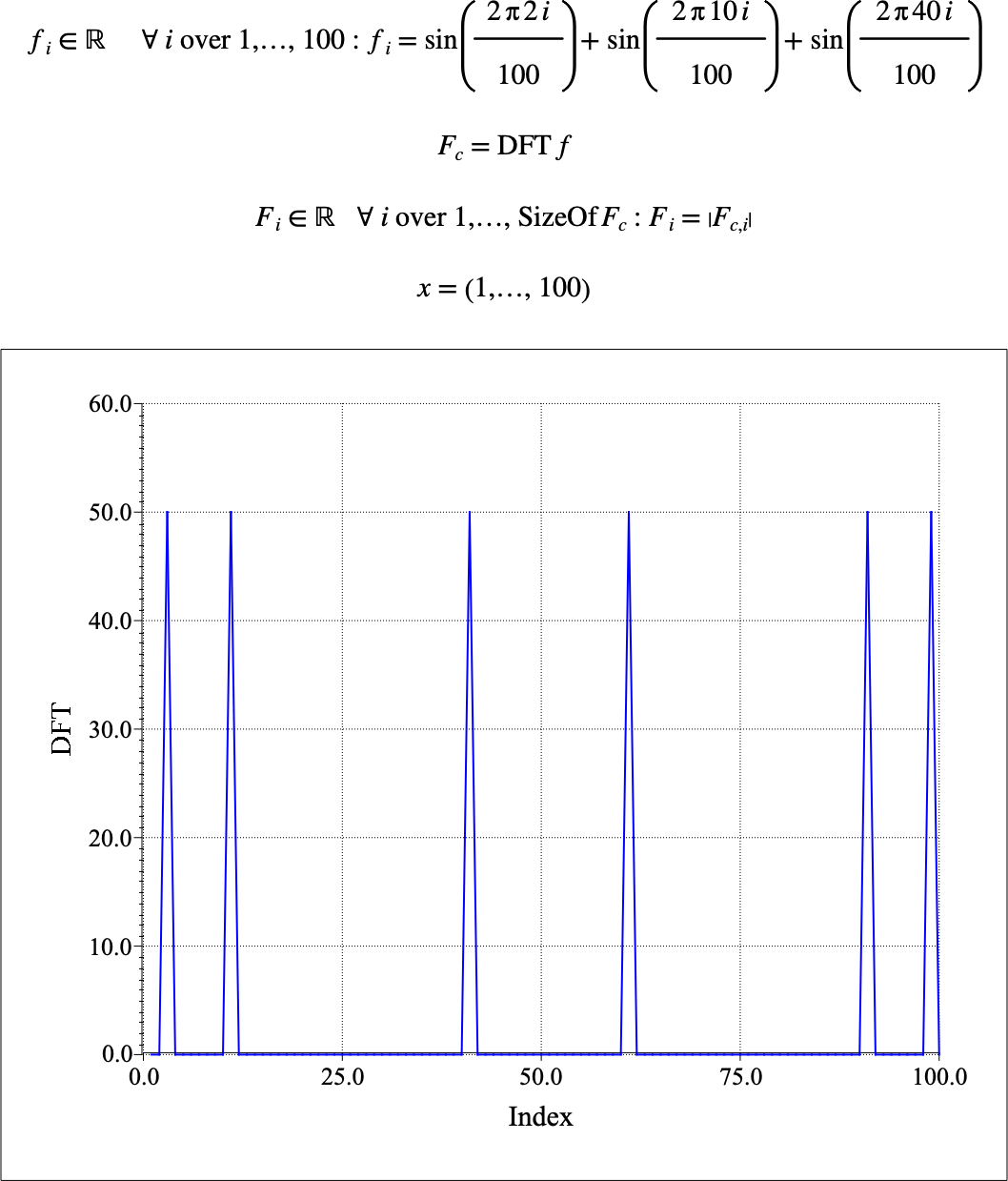# $$\text{DFT}$$¶

Calculates the discrete Fourier transform (DFT) of a matrix in one or two dimensions.

You can use the \dft backslash command to insert this function.

The following variants of this function are available:

• $$\text{complex matrix } \text{DFT} \left ( \text{<matrix>} \right )$$

The $$\text{DFT}$$ always returns a complex matrix independent of the type of the supplied input. Also note that the function assumes a cartesian representation of complex values rather than a polar representation. The returned matrix will have the same dimensions as the supplied input matrix.

This function calculates the DFT in one dimension using the relation:

$F _ { k + 1 } = - \sum_{n=0}^{N-1} f _ { n + 1 } e ^ { - \frac{2 \pi i}{N} k n }$

In two dimensions, this function calculates the DFT using the relation:

$F _ { k + 1, l + 1 } = - \sum_{m=0}^{\text{nrows} \left ( f \right ) - 1 } \sum_{n=0}^{\text{ncols} \left ( f \right ) - 1 } f _ { m + 1, n + 1 } e ^ { - 2 \pi i \left [ \frac{ k m }{ \text{nrows} \left ( f \right ) } + \frac{ l n }{ \text{ncols} \left ( f \right ) } \right ] }$

Figure 122 shows the basic use of the $$\text{DFT}$$ function.Figure 122 Example Use of The DFT Function